# Stieltjes integral

(diff) ← Older revision | Latest revision (diff) | Newer revision → (diff)

A generalization of the concept of the Riemann integral, realizing the notion of integrating a functionwith respect to another function. Let two functionsandbe defined and bounded onand let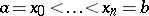. A sum of the form(1)

where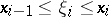,, is called a Stieltjes integral sum. A numberis called the limit of the integral sums (1) whenif for eachthere is a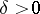such that if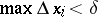, the inequality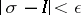holds. If the limitexists when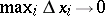and is finite, then the functionis said to be integrable with respect to the functionover, and the limit is called the Stieltjes integral (or the Riemann–Stieltjes integral) ofwith respect to, and is denoted by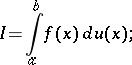(2)

the functionis said to be the integrating function. Th.J. Stieltjes  hit upon the idea of such an integral when studying the positive "distribution of masses" on a straight line defined by an increasing function, the points of discontinuity of which correspond to masses that are "concentrated at one point" .

The Riemann integral is a particular case of the Stieltjes integral, when a function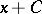, where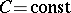, is taken as the integrating function.

When the integrating functionincreases monotonically, the upper and lower Darboux–Stieltjes sums are studied:(3)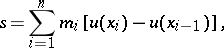where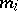and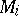are the greatest lower and least upper bounds ofon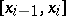.

For a Stieltjes integral to exists, it is sufficient for one of the following conditions to be fulfilled:

1) the functionis continuous on, while the functionis of bounded variation on;

2) the functionis Riemann integrable on, while the functionsatisfies a Lipschitz condition on, i.e.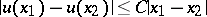, where, for any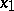andfrom;

3) the functionis Riemann integrable on, while the functioncan be represented onas an integral with a variable upper bound,whereis absolutely integrable over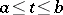.

When condition 3) is fulfilled, the integral (2) reduces to a Lebesgue integral by the formula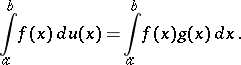(4)

(The right-hand side is a Riemann integral ifis Riemann integrable.) In particular, (4) holds ifhas a bounded and Riemann-integrable derivative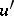on; in this case.

Ifis integrable with respect toover, thenis also integrable with respect toover. This statement leads to a number of further conditions on the existence of Stieltjes integrals.

The Stieltjes integral has the property of linearity relative to both the integrand and the integrating function (given the condition that every one of the Stieltjes integrals on the right-hand side exists):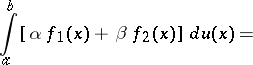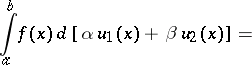Generally speaking, Stieltjes integrals do not possess the property of additivity: The existence of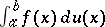does not follow from the existence of both the integrals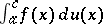and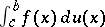(the converse is, instead, true if).

Ifis bounded on,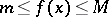, andincreases monotonically on, then there exists asatisfying the inequality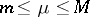, such that the mean-value formula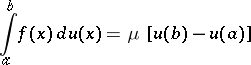(5)

holds for a Stieltjes integral. In particular, ifis continuous on, then there exists a point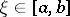such that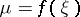.

A Stieltjes integral, whereis of bounded variation, provides the general form of a continuous linear functional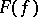on the space of continuous functions on(Riesz' theorem).

When the functionis of bounded variation, the value of the Stieltjes integral coincides with the value of the corresponding Lebesgue–Stieltjes integral.

How to Cite This Entry:
Stieltjes integral. Encyclopedia of Mathematics. URL: http://encyclopediaofmath.org/index.php?title=Stieltjes_integral&oldid=17367
This article was adapted from an original article by V.A. Il'in (originator), which appeared in Encyclopedia of Mathematics - ISBN 1402006098. See original article